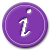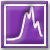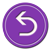# National Aeronautics and Space Administration

## Goddard Space Flight Center# Observing the Spectrum of M31Recap: Your astronomy professor has tasked the class with determining the velocity of Andromeda with respect to the Milky Way. You thought of three possible ways to do this, one of which will give you the right answer. You've decided to try using the Doppler shift of emission lines from M31's spectrum to find the velocity of M31.

You learned that the spectrum of a source can be shifted when that source is moving toward or away from you. The amount of the shift in the spectrum depends on the velocity of the source – a higher velocity results in a larger shift. The effect is called Doppler shift and is described mathematically by the following equation.

In this equation, λ' is the shifted wavelength, λ0 is the wavelength of light emitted in lab (or at rest with respect to the observer), v is the velocity of the source, and c0 is the speed of the wave in a stationary medium.Learn more about Doppler shiftLearn more about spectraUse this technique to solve for M31's velocityReturn to the beginning and try another approach